# LessonA Tale of Friction

### Quick Look

Time Required: 3 hours 15 minutes

(four 50-minute sessions)

Lesson Dependency: None

Subject Areas: Algebra, Measurement, Physics, Problem Solving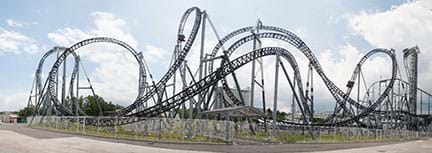Designed by engineers, roller coasters are the main attraction in theme parks around the world. No matter their scary steepness and radical movements, gravity is the force that makes them all work. One key design consideration is friction—the force that opposes movement and eventually brings the coaster cars to a stop.copyrightCopyright © 2012 Alex Brogan, Wikimedia Commons CC BY-SA 3.0 https://commons.wikimedia.org/wiki/File:Takabisha_roller_coaster.jpg

### Summary

Roller coasters projects are frequently used in middle and high school physics classes to illustrate the principle of conservation of mechanical energy. Potential energy transforms to kinetic energy and vice versa, with gravity being the driving force during the entire process. Even though friction force is mentioned, it is rarely considered in the velocity calculations along the coasters’ paths. In this high school lesson, the friction force is considered in the process. Using basic calculus and the work-energy theorem for non-conservative forces, the friction along a curved path is quantified, and the cart’s velocity along this path is predicted. This activity and its associated lesson are designed for AP Calculus. Practice problems/answers, a PowerPoint® presentation and student notes are provided. The starting point in this analysis is the solution found using the work-energy theorem to the problem of a spherical body rolling on an incline when friction is present. This approach is extended to a spherical body rolling on a curved path. Assuming that a curved path can be approximated by a sequence of many very short inclines, the problem is approached as a body rolling on this sequence of inclines, solving each with the work-energy theorem. Defining the curved path as a differentiable function, the slope of each incline is obtained through the function derivative. Formulas for the coefficient of static friction, friction force and velocity are found and through them, values of these properties along the curved path can be determined. Students use these equations in the associated activity to design and construct simple roller coasters that consider the friction present, using a flexible material like foam pipe insulation as the coaster’s path and a marble as the cart.
This engineering curriculum aligns to Next Generation Science Standards (NGSS).

### Engineering Connection

With the costs to create roller coasters ranging from \$20 to 100 million (R1), engineers must be scrupulous in their designs and consider myriad details before construction begins because mistakes have high costs, or even worse, terrible accident consequences (R2). But no matter a coaster’s complexity and cost, all have something in common—gravity and inertia propels the carts along the entire path. Potential energy transforms into kinetic energy and vice versa. Another key force that affects roller coasters is friction—the force that gradually decelerates the carts until it practically stops them. Engineers who design roller coasters aim to optimize the kinetic-potential energies and minimize friction effects.

In this lesson, potential-kinetic energies and friction force are quantified along a simple curved path. Using basic calculus and the work-energy theorem for non-conservative forces, a formula to find the velocity of a spherical body rolling along a curved path is determined. This basic equation enables students to design simple roller coasters and know before construction whether their designs will work or not—just like roller coaster engineers do in real projects.

### Learning Objectives

After this lesson, students should be able to:

• Estimate the effect of friction for a spherical body rolling on an incline.
• Use free-body diagrams to analyze the friction force acting on a body rolling on an incline.
• Use the work-energy theorem for non-conservative systems to quantify the work done by friction forces.
• Quantify the coefficient of static friction for a spherical body rolling along a variable slope path.
• Quantify the static friction for a spherical body rolling along a variable slope path.
• Determine the linear velocity of a spherical body rolling along a variable slope path with friction.

### Educational Standards Each TeachEngineering lesson or activity is correlated to one or more K-12 science, technology, engineering or math (STEM) educational standards. All 100,000+ K-12 STEM standards covered in TeachEngineering are collected, maintained and packaged by the Achievement Standards Network (ASN), a project of D2L (www.achievementstandards.org). In the ASN, standards are hierarchically structured: first by source; e.g., by state; within source by type; e.g., science or mathematics; within type by subtype, then by grade, etc.

###### NGSS: Next Generation Science Standards - Science
• Analyze complex real-world problems by specifying criteria and constraints for successful solutions. (Grades 9 - 12) More Details

Do you agree with this alignment?

###### Common Core State Standards - Math
• (+) Use special triangles to determine geometrically the values of sine, cosine, tangent for π/3, π/4 and π/6, and use the unit circle to express the values of sine, cosine, and tangent for π-x, π+x, and 2π-x in terms of their values for x, where x is any real number. (Grades 9 - 12) More Details

Do you agree with this alignment?

• Calculate and interpret the average rate of change of a function (presented symbolically or as a table) over a specified interval. Estimate the rate of change from a graph. (Grades 9 - 12) More Details

Do you agree with this alignment?

• Graph functions expressed symbolically and show key features of the graph, by hand in simple cases and using technology for more complicated cases. (Grades 9 - 12) More Details

Do you agree with this alignment?

###### International Technology and Engineering Educators Association - Technology
• Illustrate principles, elements, and factors of design. (Grades 9 - 12) More Details

Do you agree with this alignment?

###### Texas - Math
• apply mathematics to problems arising in everyday life, society, and the workplace; (Grades 9 - 12) More Details

Do you agree with this alignment?

• analyze mathematical relationships to connect and communicate mathematical ideas; and (Grades 9 - 12) More Details

Do you agree with this alignment?

• analyze situations modeled by functions, including exponential, logarithmic, rational, polynomial, and power functions, to solve real-world problems; (Grades 9 - 12) More Details

Do you agree with this alignment?

• determine the value of trigonometric ratios of angles and solve problems involving trigonometric ratios in mathematical and real-world problems; (Grades 9 - 12) More Details

Do you agree with this alignment?

• use trigonometry in mathematical and real-world problems, including directional bearing; (Grades 9 - 12) More Details

Do you agree with this alignment?

###### Texas - Science
• calculate the effect of forces on objects, including the law of inertia, the relationship between force and acceleration, and the nature of force pairs between objects; (Grades 9 - 12) More Details

Do you agree with this alignment?

• develop and interpret free-body force diagrams; and (Grades 9 - 12) More Details

Do you agree with this alignment?

• investigate and calculate quantities using the work-energy theorem in various situations; (Grades 9 - 12) More Details

Do you agree with this alignment?

• investigate examples of kinetic and potential energy and their transformations; (Grades 9 - 12) More Details

Do you agree with this alignment?

• calculate the mechanical energy of, power generated within, impulse applied to, and momentum of a physical system; (Grades 9 - 12) More Details

Do you agree with this alignment?

• demonstrate and apply the laws of conservation of energy and conservation of momentum in one dimension; (Grades 9 - 12) More Details

Do you agree with this alignment?

Suggest an alignment not listed above

### Pre-Req Knowledge

A basic understanding of slope of a line, right triangle trigonometry, differentiable functions, derivative of a function, linear velocity and acceleration, linear kinetic and potential energy, rotational kinetic energy and moment of inertia of rigid body, static friction, free-body diagrams, and work-energy theorem.

### Introduction/Motivation

(Be ready to show the 21-slide A Tale of Friction Presentation, a PowerPoint® file, including some short online videos. The slides are animated, so a mouse or keyboard click brings up the next text, image and/or slide. Begin the lesson by playing some of the videos linked on slide 2. Then begin a class discussion using the prompts provided below.)

Do you like roller coasters? Have you ever ridden one? (Expect several students to raise their hands. Ask those not raising their hands why they have not ridden one. Some answers may be: it’s scary, or the speeds and drops/falls make me feel sick.)

Have you ever thought about all the work necessary to design and construct these scream machines? What do you imagine are some of the steps in this process? (Listen to some answers. Expect some to include “design” and “construction.”) Roller coasters design and construction are challenging tasks for engineers. They are also expensive projects in which errors and/or omissions may cause losses of money or terrible accidents (R2).

No matter how complex the design, the main force that makes all coasters work is gravity. Potential energy transforms to kinetic energy, and coaster carts can move up to 90 mph, like we saw in the video for Intimidator305, at Kings Dominion theme park in Virginia (see Figure 1).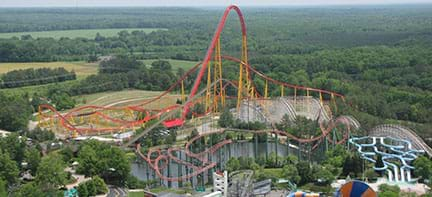Figure 1. Costing \$25 million to create, the one-mile-long Intimidator 30 roller coaster in Virginia reaches a maximum velocity of 91 mph and its carts drop 300 feet.copyrightCopyright © 2011 Ben Schumin, Wikimedia Commons CC BY-SA 3.0 https://commons.wikimedia.org/wiki/File:Intimidator_305_ride_as_seen_from_Eiffel_Tower.jpg

But we know that those velocities decrease as carts move along the coaster track. Friction forces are the cause of this deceleration. To create the fastest and longest rides possible, engineers must quantify friction at every point of the coaster’s path, and make design choices to counteract that friction.

Do you have any ideas about how engineers do this? Remember that friction is a dissipative force, which means that in this case kinetic energy transforms into other energy forms, such as heat. As an example, rub your hands together. (Expect students to provide the answer: Engineers do this by using energy conservation principles.)

Many physics problems are solved using the concept of conservation of energy. In this lesson, you will learn the same basic physics approach that real-world engineers use to design roller coasters that take into consideration friction forces. With the equations you learn, you will be able to design a simple roller coaster—we’ll call it Russian Mountain—and know before it is even built the velocity of a body (cart) rolling along its route, as well as the friction forces acting on it.

### Lesson Background and Concepts for Teachers

This lesson and its associated activity is suitable for the end of the first semester of the school year for high school AP Calculus courses, serving as a major grade for the last six-week period with the results presentation-report (see the associated activity Mathematically Designing a Frictional Roller Coaster) taken as the first-semester finals test.

Provided in this section is the detailed process to obtain the formulas to predict velocities and friction forces for a spherical body rolling along a curved path. Parts 1 and 2 contain basic concepts students usually cover in a pre-AP Physics course. Parts 3 and 4 are extensions of these concepts—and the core of this lesson. The same information is provided in Student Notes as a 10-page student handout.

1.0 Basic Concepts

1.1 Rotational Movement Kinematics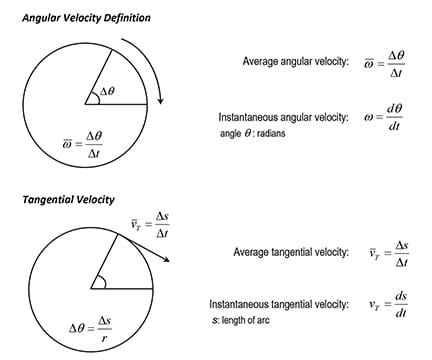Figure 2. Similar to how linear velocity is defined, angular velocity is the angle swept by unit of time. Tangential velocity is equivalent to linear velocity for a particle moving on a circumference.copyrightCopyright © 2017 Miguel R. Ramirez

Because θ=s/r (Figure 2), (r = circle radius, s = arc length), s = θ * r, then the angular and tangential velocities are related as:

If angular acceleration is defined as: α = dω/dt, and tangential acceleration as: aT = dvT/dt, then,

The angular and tangential accelerations are then related as: aT = α * r.

1.2 Rotational Kinetic Energy and Moment of Inertia of a Rigid Body

For a single particle, the kinetic energy for linear movement is defined as K = ½ mv2. Similarly, for a single particle, the kinetic energy for rotational movement is given by the formula K = ½ Iω2, where I = mr2 is known as moment of inertia of the particle, and r is the distance from the point of rotation.

For a system of particles, its moment of inertia is the sum of the individual moments I = ∑miri2. This definition can be extended to compute the moment of inertia for a continuous rigid body:

For the special case analyzed in this project, the moment of inertia for a solid homogeneous sphere is:

1.3 Angular Momentum and Torque of a Rigid Body

From the law of lever, the torque, or amount of rotation produced by a force, is mathematically defined as τ=F*d, where F is the perpendicular force applied on the lever at a distance d from the fulcrum. Extending this definition, taking the force F as a vector F non-perpendicular to the vector  that gives the position of the point where the force is applied with respect to the rotation point, the torque is defined as the vector product:

where the torque τ is represented as a vector perpendicular to the plane (Figure 3) defined by vectors r  and F. The magnitude of this vector is given by the product of the magnitudes of the vectors r and F, (r and F) times the sine of the angle between them:

When the angle is 90o, r and F are perpendicular, and the original law of lever is obtained.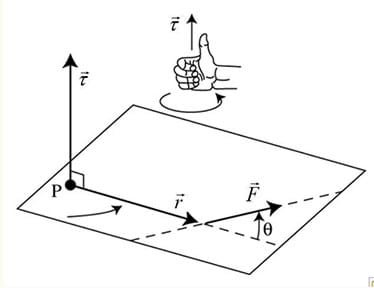Figure 3. Torque is a measure of how much a force acting on an object causes that object to rotate. It is defined as a vector coming from the special product of the position vector for the point of application of the force, and the force vector. Its magnitude depends on the angle between position and force vectors. If these vectors are parallel, the torque is zero.copyrightCopyright © 2017 Miguel R. Ramirez

For a single particle, the linear momentum is defined as the vector: = m * v , and by definition, the force or Newton’s second law for a single particle when its mass changes with the time is given by:

When the mass of the particle is constant:

Similar to the linear momentum, the angular momentum of a particle is defined as:

and similar to the force definition for linear movement in terms of the linear momentum, the force producing a rotational movement, or torque, is defined as:

For a particle of constant mass in a circular movement (r constant), and v, the tangential velocity (θ = 90o), the magnitude of the torque is given by:

a formula similar to Newton’s second law for a rotating rigid body with constant mass.

2. Force of Friction for a Spherical Rigid Body Rolling without Slipping on an Incline Surface

A spherical rigid body of mass m is rolling down an incline surface. The forces producing this movement are the weight of the body mg and the force of friction fs (Figure 4).

Because the body is rolling instead sliding, the friction to consider in this problem is static friction. So, the coefficient to use in the friction force calculations is the static friction coefficient μs.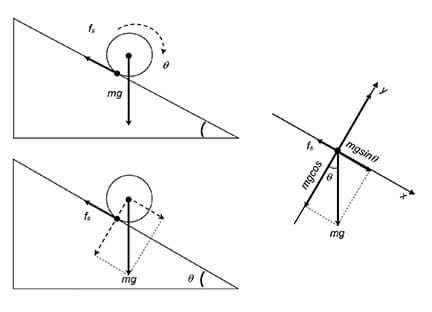Figure 4. Free-body diagrams are widely used in physics to show the relative magnitude and direction of all forces acting upon objects in given situations. For this problem, a body’s weight and surface friction are the forces acting on a spherical body rolling on an incline.copyrightCopyright © 2017 Miguel R. Ramirez

The body rolls because a torque is produced by the friction force fs and the component of the body’s weight parallel to the inclined surface (Figure 4). All forces on the rolling body can be analyzed by using a free-body diagram.

The forces that make this spherical solid of radius r and mass m roll down the incline plane are those along the x-axis. Using Newton’s second law to describe the solids linear movement:

The force that makes the solid rotate is the torque produced by the friction force:

where I = sphere’s momentum of inertia, α = sphere’s angular acceleration, r = sphere’s radius for a homogenous spherical object: I = (2/5)*m*r2. Substituting this value, and α = a/r in (2):

The force of friction can be expressed as:

Substituting (3) in (1):

Substituting the obtained acceleration value in (3), the force of friction can be expressed in terms of the sphere’s weight and the angle of the incline:

By definition, the static friction force is proportional to the normal force, or force the surface applies on the sphere to balance the component of the sphere’s weight perpendicular to the surface.

where μs is the static friction coefficient and Fn is the magnitude of the normal force. For this problem, the value of the normal force can be found in the free-body diagram:

Substituting this value in (5):

Combining equations (4) and (6), an expression for the coefficient of static friction can be found:

Equation (7) states that for a spherical body rolling on an incline, the coefficient of static friction is a function of the inclined surface angle only, specifically, of the tangent of this angle or slope of the inclined surface.

3. Force of Friction for a Spherical Rigid Body Rolling without Slipping on a Variable Slope Path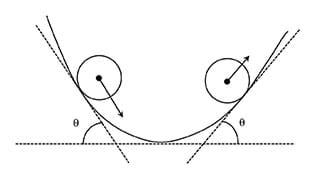Figure 5. At any point of a curved path f (x), the tangent lines can be visualized as portions of an incline. The slope of the incline is the tangent of the angle between this line and the horizontal. In calculus, this slope is given by the value of the derivative of the function f (x) at that point.copyrightCopyright © 2017 Miguel R. Ramirez

A sphere rolling on a path with variable slope can be visualized as rolling on a sequence of inclined tangent straight surfaces. If the path is given as a function y = f (x), differentiable at every point, the slope of any these inclines, defined as m = tan θ (Figure 5), can be found using the derivative f ’(x):

Then:

Using the above expression in (7), the coefficient of static friction for a sphere rolling on a variable slope path is given by:Expression (8) states that the static friction coefficient varies from point to point on the path. Using equation (8) in (6), the static friction force for a rolling sphere of mass m is:

Using trigonometry, it is possible to express cos θ also in terms of f ’(x). Because tan θ = f ’(x), then:

Then:

Solving with right triangles (Figure 6):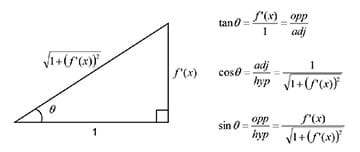Figure 6. The slopes of the tangent lines of a function f (x), can be calculated using the derivative of the function, f ’(x). Using right triangle trigonometry, it is possible to express the cosine of the angles of these lines also in terms of the derivative of the function. copyrightCopyright © 2017 Miguel R. Ramirez

Using this expression for cos θ in (9):

Equations (8) and (10) seem to be adequate to model the problem of a sphere rolling on a variable slope path with friction, but something needs to be fixed. Both equations give negative values for some sections of the path, and by definition, the static friction coefficient is always positive.

The problem comes from the sign of the derivative and the possibility of negative slopes. For the inclined plane, the elevation angle is always positive. Because in this problem we approached the path with variable slope as a sequence of inclines, the slope given by f ’(x) must always be positive. With this restriction, equations (8) and (10) must be given in terms of the absolute value of f ’(x):

4. Work-Energy Theorem for a Sphere Rolling on a Variable Slope Path with Friction

The work-energy theorem states that the mechanical energy (kinetic energy + potential energy) of an isolated system under only conservative forces remains constant.

where E is the mechanical energy of the system, K is the kinetic energy ½ mv2, U is the potential energy mgh, and the indexes f and i indicate the energies at the end and beginning of the process, respectively.

While energy cannot be created nor destroyed in an isolated system, it can be internally converted to other energy forms.

When a non-conservative force like friction is considered in a system, the work-energy theorem states that the work done by the non-conservative forces, ΔWf, is equivalent to the change in mechanical energy:

Under non-conservative forces, ΔE is no longer zero, but another key difference exists. Meanwhile for conservative systems, the work done by conservative forces depends only on the initial and final positions. For non-conservative systems, the work done by non-conservative forces, like friction, depends on the path or trajectory, or on the time these forces affect the system. In a system under conservative forces, the work on a closed loop is always zero, while in a system under non-conservative forces it is not.

By definition, mechanical work is the product of the displacement times the force component along the displacement (Figure 7):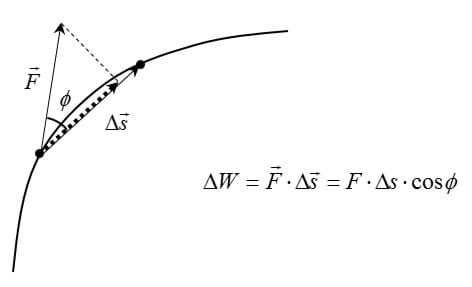Figure 7. Mechanical work is done when a force that is applied to an object moves that object, and it is defined as the product of the force value and the displacement of the object. Work is done only by the component of the force parallel to the displacement produced.copyrightCopyright © 2017 Miguel R. Ramirez

For the variable slope path y = f (x), the work done by the force of friction (12) is:

Taking differential displacements along the path:

If the differential arc ds is defined as:

the arc ds can be set as a function of f ’(x):

Substituting in (15) in (14):

Because dx > 0, |dx| = dx, then:

Because the friction force always acts against the movement, the work done by friction is always negative. Then:

Again taking small displacement Δs along the path:

For the entire path, the total work is the sum of the work done along the little displacements the path had been divided into (Figure 8).

Substituting equation (16) in (13):

Then:

because the height of the object on the path is given by the value of function f (x) (that is, h = f (x)).

Using the above equation, it is possible to find the velocity of the rolling body at the end of every little displacement (Figure 8), knowing the values of its velocity at the beginning of the displacement, and of the corresponding potential energy change: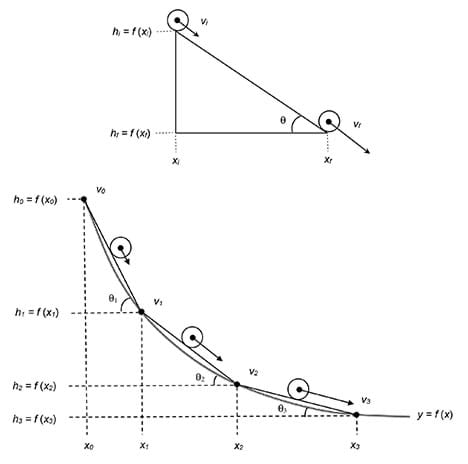Figure 8. The rolling of a spherical body on a curved path can be approximated as the rolling of this body on a sequence of inclines. The final velocity at the end of one incline is the initial velocity at the beginning of the next incline.copyrightCopyright © 2017 Miguel R. Ramirez

Teacher Note

The purpose of this lesson is to provide the necessary physics background so students understand why equation (18) is useful in the design of a roller coaster, which they need in order to complete the associated activity, Mathematically Designing a Frictional Roller Coaster. If time is limited, you may want to skip Sections 1 and 2, and assign students, as homework, to watch the online tutorial videos about the topics of friction, and rotational dynamics (S1). Be sure students fully understand these concepts, or it will be difficult for them to understand the next ones.

Since no online tutorials are available for Sections 3 and 4, which are the core lesson concepts, they must be covered by the teacher in class. Use the A Tale of Friction Presentation to help in the explanation of these important concepts, and hand out the Student Notes. Having these 10 pages of notes in hand frees students to pay more attention to the teacher explanations. Inform students of the importance of these concepts to completing the hands-on activity they will engage in later, and alert them that you will lecture them as a college professor would. As makes sense for your students, verify they understand what you are explaining. The Practice Problems are provided as one way to assess student comprehension.

Become familiar with all the concepts and mathematical steps in this lesson. Feel free to contact the author for clarification of any of the above depicted steps.

The suggested sequence to conduct the lesson is:

Day 1. (optional) Basic Concepts

Section 1.1: Rotational Movement Kinematics

Section 1.2: Rotational Kinetic Energy and Moment of Inertia of a Rigid Body

Section 1.3: Angular Momentum and Torque of a Rigid Body

Day 2. (optional) Friction

Section 2: Force of Friction for a Spherical Rigid Body Rolling without Slipping on an Incline Surface

Day 3. Rolling with Friction A

Section 3: Force of Friction for a Spherical Rigid Body Rolling without Slipping on a Variable Slope Path

Day 4. Rolling with Friction B

Section 4: Work-Energy Theorem for a Sphere Rolling on a Variable Slope Path with Friction

### Associated Activities

• Designing a Frictional Roller Coaster With Math and Physics! - Students use the approach and formulas learned in this lesson to design simple 2D roller coasters—Russian Mountains—creating the paths with differentiable functions. Each team determines the velocities and friction forces along its coaster path and mathematically proves that its design works. After creating coaster paths, teams use foam pipe insulation attached to cardboard backing to construct prototype models of the designed roller coasters, and then test them using marbles as the carts.

### Lesson Closure

1. Ask students to write down on a piece of scratch paper a brief explanation of why the work-energy theorem was used to solve the problem of a solid rolling on an incline when friction is present. (Correct answers incorporate the concept that friction force does work on a body because it opposes movement, and according to the work-energy theorem, the work done on the body equals the change in its mechanical energy.)
2. Ask students to briefly explain how the problem of a solid rolling on a curved path was approached. (Correct answers include the concept that a complex curve can be approximated by many short straight connected segments, and because of this, the complex problem of a body rolling on a curved path, can be approached by the same body rolling on many short simple consecutive inclines.)

### Vocabulary/Definitions

acceleration: The change in the velocity of an object. The average acceleration is defined as the change in velocity divided by time. The instantaneous acceleration is defined as the acceleration at any particular time period. In calculus, given the velocity of a body as a function of the time, the instantaneous acceleration is the derivative if the velocity with respect to the time.

continuous function: In a very basic approach, a function whose graph does not have any void and/or it is not broken, that is, can be drawn without lifting the pencil from the paper. More formally, a function is continuous at a point in its domain if a sufficiently small change in the input results in an arbitrarily small change in the output. In calculus, a function is continuous at a point x = c if and only if all the next three conditions are met: 1) the function is defined at x = c, 2) the limit of the function at x = c exists, and 3) the limit and the value of the function at x = c are equal.

derivative: The limit of the ratio of the change in a function to the corresponding change in its independent variable as the latter change approaches zero. Geometrically, this rate of change gives the slope of the tangent line at a point on the function’s graph. The graph of the function at that point must be continuous and smooth, that is, the function cannot have a peak at this point.

differentiable function : A function whose derivative exists at each point in its domain. Geometrically, the function whose graph is continuous and smooth such that a tangent line exists for every point on the graph.

energy conservation: A principle that states that in a system that does not undergo any force from outside the system, the amount of energy is constant, irrespective of its changes in form.

friction: The resistance to motion of one object moving relative to another. The surface resistance to relative motion, as of a body sliding or rolling. The force resisting the relative motion of solid surfaces, fluid layers, and material elements sliding against each other.

gravitational force: The force of attraction between masses; the attraction of the earth’s mass for bodies near its surface. The gravitational force between two bodies is proportional to the product of their masses and inversely proportional to the square of the distance between them.

inertial force: A force that resists a change in velocity of an object. It is equal to the applied force, but with opposite direction. Because of this force, every object in a state of uniform motion, or rest, tends to remain in that state unless an external force is applied to it.

kinetic energy: The energy possessed by an object due to its motion or movement. It is proportional to its mass and to the square of its velocity. The magnitude of this energy arises from the net work done on the object, to accelerate it from rest to its final velocity. Kinetic energy can be transformed again in work.

kinetic friction: A force that acts between moving surfaces. It is also known as sliding friction or moving friction, and it is the amount of retarding force between two objects that are moving relative to each other.

maximum of a function: The largest value of a function, either within a given range or on the entire domain. Formally, a function f (x) has a maximum at x = c, a < c < b, [a, b] a subinterval of the function’s domain, if f I > f (x), for a ≤ x ≤ b.

mechanical energy: The capacity of a physical system to change an object’s state of motion.

mechanical work: The amount of energy transferred by a force that moves an object. The work is calculated by multiplying the applied force by the amount of movement of the object.

minimum of a function: The smallest value of a function, either within a given range or on the entire domain. Formally, a function f (x) has a minimum at x = c, a < c < b, [a, b] a subinterval of the function’s domain, if f I< f (x), for a ≤ x ≤ b.

parabola: A conic section formed by the intersection of a vertical cone by a plane parallel to the cone’s side. A curve where any point is at an equal distance from a fixed point, the focus, and a fixed straight line, the directrix.

parabola vertex: The point where a parabola crosses its axis of symmetry. It is the maximum point when the parabola opens downwards, or the minimum point if the parabola opens upwards.

piecewise function: A function that is defined by multiple sub-functions, each sub-function applying to a certain interval of the main function’s domain. Also known as a hybrid function.

potential energy: The energy possessed by a body by virtue of its position relative to others. In a gravitational field, potential energy is the energy stored in an object as the result of its vertical position or height. The energy is stored as the result of the gravitational attraction of the earth for the object.

roller coaster: An amusement park ride that consists on an elevated railroad track designed with sharp curves and steep slopes on which people move in small, fast and open rolling cars.

Russian Mountains: The oldest roller coaster design and a predecessor to the modern day roller coaster. Descended from Russian winter sled rides on hills of ice, these early roller coasters were open wheeled carts or open train carts on tracks of elevated up-hills and down-hills supported by wooden or steel structures.

secant line: The straight line joining two points on a curve.

static friction: The friction that exists between a stationary object and the surface on which it rests—the force that keeps an object at rest and that must be overcome to start moving an object. Pushing horizontally with a small force, static friction establishes an equal and opposite force that keeps the object at rest.

tangent line: The straight line that touches a curve at a point without crossing over.

velocity: The time rate of change of position of a body in a specified direction. The average velocity is defined as the change in position divided by the time of travel. The instantaneous velocity is simply the average velocity at a specific instant in time. In calculus, given the position of a body as a function of the time, the instantaneous velocity is the derivative of the position with respect to the time.

work-energy theorem: A principle that states that the work done by all forces acting on a particle equals the change in the particle’s kinetic energy.

### Assessment

Pre-Lesson Assessment

Review: Verify that students understand physics concepts such as gravity, kinetic and potential energy, energy conservation, velocity, acceleration, force and friction. Also verify that they understand math concepts like slope, differentiable function (smooth curves), derivative and tangents to curves, and right triangle trigonometric functions. Do this via the method most effective with your students, such as a short quiz, warm-up exercise, or brief discussion. Consider assigning students, as homework, to watch the online tutorial videos about the topics of friction, and rotational dynamics provided in the author’s Sophia.org AP Calculus First Semester Project playlist (S1).

Post-Introduction Assessment

Discussion: After the introduction, begin a short general class discussion asking questions such as the following. Write students’ answers on the board and briefly discuss them. Also direct students to search on the web for the scariest roller coasters and note how fast they can go and any related interesting information.

• Why is physics important in engineering?
• What are some examples of how physics concepts are used in engineering?

Lesson Summary Assessment

Practice Problems: To verify whether or not students understand how to use the obtained formulas and concepts, have students complete the Practice Problems. Review their answers to assess their depth of comprehension.

As part of the introduction, show students some of these roller coaster videos linked on slide 2:

Lesson support AP Calculus First Semester Project online tutorials for students at Sophia (by the author; S1): https://www.sophia.org/playlists/ap-calculus-first-semester-project, on the following topics:

• Friction concepts and solutions for the problem of a body rolling on a surface with friction
• Rotational kinematics and dynamics
• Piecewise differentiable functions

### Subscribe

Get the inside scoop on all things TeachEngineering such as new site features, curriculum updates, video releases, and more by signing up for our newsletter!
PS: We do not share personal information or emails with anyone.

### More Curriculum Like This

High School LessonDoing the Math: Analysis of Forces in a Truss Bridge

Learn the basics of the analysis of forces engineers perform at the truss joints to calculate the strength of a truss bridge known as the “method of joints.” Find the tensions and compressions to solve systems of linear equations where the size depends on the number of elements and nodes in the trus...

High School LessonMay the Magnetic Force Be with You

After a demonstration of the deflection of an electron beam, students review their knowledge of the cross-product and the right-hand rule with example problems. Students apply these concepts to understand the magnetic force on a current carrying wire. Through the associated activity, students furthe...

### Other Related Information

You are welcome to contact the author, Miguel R. Ramirez, at mramirez2@galenaparkisd.com or 832-386-2922 to ask any questions related to this activity.

### Contributors

Miguel R. Ramirez, Galena Park High School

### Supporting Program

Miguel R. Ramirez, high school math teacher, Texas, USA

### Acknowledgements

The author expresses his thanks to Tony Gardea, Galena Park High School Principal; Seretha Augustine, Galena Park High School Associate Principal for Curriculum; and Gerard Kwaitkowski, Galena Park High School Math Specialist.